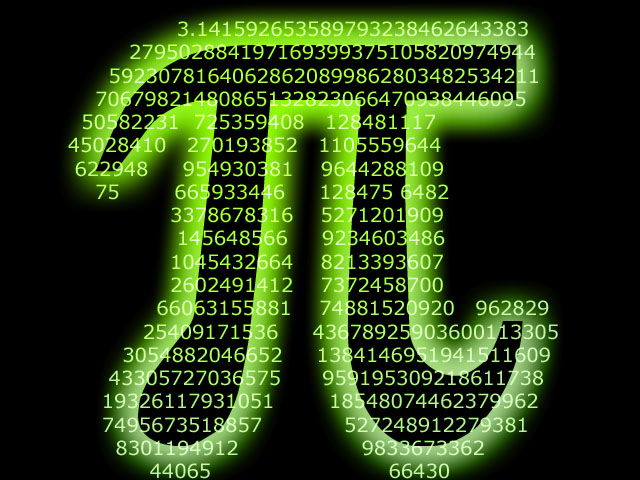#Physical Constants (SI Units)

Avogadro Constant$N_{A} = 6.022 \times 10^{23} mol^{-1}$

Bohr Radius$a_0 = 5.29 \times 10^{-11} m$

Boltzmann Constant$k = 1.381 \times 10^{-23} JK^{-1}$

Charge on an Electron$e = - 1.602 \times 10^{-19} C$

Gravitational Constant$G = 6.673 \times 10^{-11} Nm^{2} kg^{-2}$

Magnitude of Acceleration due to Gravity$g = 9.81 m s^{-2}$

Permeability of Free Space$\mu_{0} = 4 \pi \times 10^{-7} T m A^{-1}$

Permittivity of Free Space$\epsilon_{0} = 8.854 \times 10^{-12} C^{2} N^{-1} m^{-2}$

Speed of Light in a vacuum$c = 2.998 \times 10^{8} m s^{-1}$